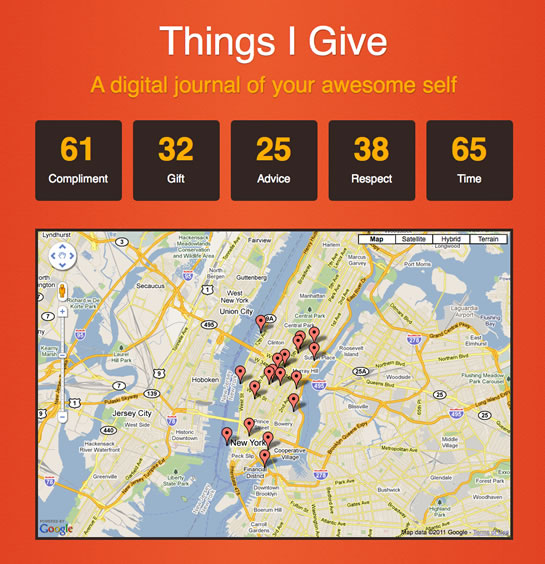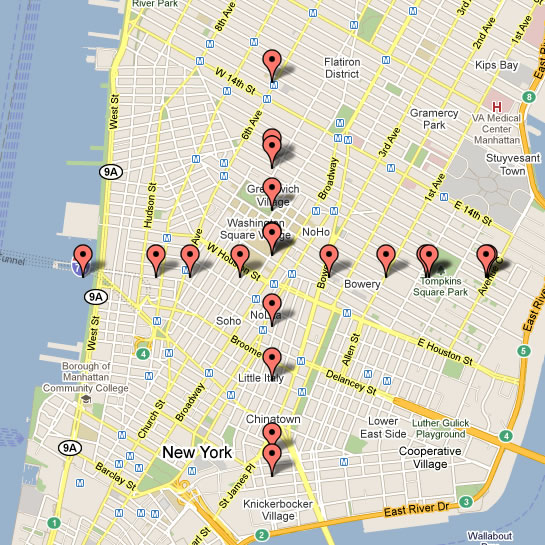On User Experience (UX) Design, JavaScript, ColdFusion, Node.js, Life, and Love.

# Creating Fuzzy Latitude And Longitude Values With A Given Amount Of Freedom

By on

Recently, I added location tracking to the Things-I-Give web application. As I talked about before, ThingsIGive.com allows users to track their own good deeds in an effort to "gamify" the act of giving; the hope, of course, being that users will become greedy for giving (built in an effort to combat my own anxiety about giving). Although the web application is completely anonymous, I know that people are still a bit weary of giving out location information. As such, I wanted to take the global position reported by the web application and make it a bit "fuzzy."When the browser reports its location, either through the Javascript Geolocation API or its remote IP address, the location is defined as latitude and longitude coordinates. These are given in degrees. I wanted to create a function that would take a latitude-longitude point and a given "miles of freedom" and then return a random position based on the miles of freedom.

Doing this simply required a little math to figure out how many miles-per-degree were available at the given latitude and longitude. Here's what I came up with:

• <cffunction
• name="fuzzyLatLong"
• access="public"
• returntype="struct"
• output="false"
• hint="I make the given latitude and longitude values a tiny bit fuzzy depending on the given miles of freedom.">
•
• <!--- Define arguments. --->
• <cfargument
• name="latitude"
• type="numeric"
• required="true"
• hint="I am the Float value indicating one of the latitdue degree value."
• />
•
• <cfargument
• name="longitude"
• type="numeric"
• required="true"
• hint="I am the Float value indicating one of the longitude degree value."
• />
•
• <cfargument
• name="miles"
• type="numeric"
• required="false"
• default="5"
• hint="I am the number of miles of 'fuzziness' that we we'll use in our latitude / longitude randomization."
• />
•
• <!--- Define the local scope. --->
• <cfset var local = {} />
•
• <!---
• Get the number of miles per degree. This is only an estimate
• based on latitude. The longitude will have to be determined
• based on teh latitude.
• --->
• <cfset local.milesPerDegreeLatitude = 69.09 />
•
• <!---
• Now that we have the miles per degree of latitude, we need to
• figure out what the same distance is for the longitude at the
• given latitude.
•
• NOTE: This forumla was taken from the following Google
• --->
• <cfset local.milesPerDegreeLongitude = (local.milesPerDegreeLatitude * cos( arguments.latitude * pi() / 180 )) />
•
• <!---
• Determine the number of degrees that we will need to
• randomize the latidude based on the miles of freedom.
• --->
• <cfset local.degreesOfLatitudeRandomness = (arguments.miles / local.milesPerDegreeLatitude) />
•
• <!---
• Determine the number of degrees that we will need to
• randomize the longitude based on the miles of freedom.
• --->
• <cfset local.degreesOfLongitudeRandomness = (arguments.miles / local.milesPerDegreeLongitude) />
•
• <!---
• Create a struct to hold the fuzzy version of the
• latitude and longitude values. For each of these values,
• we'll subtract the maximum randominess then add a fraction
• of 2x the randomness. This will give us a random value
• between our negative random max and our positive random max.
• --->
• <cfset local.coordinates = {
• latitude = (
• (arguments.latitude - local.degreesOfLatitudeRandomness) +
• (
• local.degreesOfLatitudeRandomness *
• (2 * rand( "sha1prng" ))
• )
• ),
• longitude = (
• (arguments.longitude - local.degreesOfLongitudeRandomness) +
• (
• local.degreesOfLongitudeRandomness *
• (2 * rand( "sha1prng" ))
• )
• )
• } />
•
• <!--- Return the resultant coordinate value. --->
• <cfreturn local.coordinates />
• </cffunction>
•
•
• <!--- ----------------------------------------------------- --->
• <!--- ----------------------------------------------------- --->
•
•
• <cfoutput>
•
• <!DOCTYPE html>
• <html>
• <title>Creating Fuzzy Latitude And Longitude Values</title>
• <body>
•
• <!--- Our map container. --->
• <div id="map" style="height: 950px ;"></div>
•
• <script type="text/javascript">
•
• // Set up the Google map stuff.
• var mapContainer = document.getElementById( "map" );
•
• var map = new google.maps.Map(
• mapContainer,
• {
• zoom: 13,
• 40.725665,
• -73.996353
• ),
• }
• );
•
•
• // I add maker to the map and initialize it such that it
• // will respond to click events.
• var addMarkerToMap = function( latLong ){
• // Create new marker from the location.
• var marker = new google.maps.Marker({
• map: map,
• position: latLong
• });
•
• // Return the newly created marker.
• return( marker );
• };
•
•
• // ---------------------------------------------- //
• // ---------------------------------------------- //
•
•
• <!--- Add vertical markers. --->
• <cfloop index="i" from="1" to="10">
•
• <!---
• Get our fuzzy position with a fuzzy freedomness
• of one mile.
• --->
• <cfset position = fuzzyLatLong(
• 40.725665,
• -73.996353,
• 1
• ) />
•
• <!--- Keep the longitude constant. --->
• #position.latitude#,
• -73.996353
• )
• );
•
• </cfloop>
•
•
• <!--- Add horizontal markers. --->
• <cfloop index="i" from="1" to="10">
•
• <!---
• Get our fuzzy position with a fuzzy freedomness
• of one mile.
• --->
• <cfset position = fuzzyLatLong(
• 40.725665,
• -73.996353,
• 1
• ) />
•
• <!--- Keep the latitude constant. --->
• 40.725665,
• #position.longitude#
• )
• );
•
• </cfloop>
•
• </script>
• </body>
• </html>
•
• </cfoutput>

As you can see, this function, fuzzyLatLong(), essentially determines how many degrees of freedom there are at the given location based on the given number of "fuzzy" miles. Once the degrees of freedom have been found, it then becomes a matter of randomly selecting a point between the -MAX and MAX degree values.

When we run the above page, we get the following Google Maps output:As you can see, these points have been randomly distributed within a mile of the original position (where the two lines intersect). In this case, I have only randomized one of the coordinates in order to illustrate the distribution. However, when put into practice, both the latitude and longitude would be randomized.We do something similar to mask real estate values for agents who wish to not display the exact address... Our geocodes would ruin that, so we randomize it within .05 miles, which ends up being 1-2 blocks.

We also make it a polygon circle to encompass that address. Pff. Real Estate :P